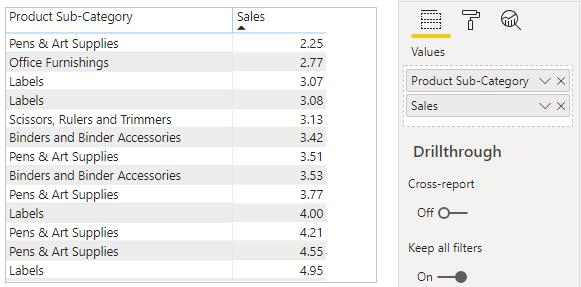Home » DAX » DAX – MIN, MINA & MINX Functions

# DAX – MIN, MINA & MINX FunctionsPower BI DAX functions MIN, MINA & MINX are aggregation functions and returns the smallest value in a column. All functions comes under statistical functions Dax categories.

### 1- MIN DAX Function:

Returns the smallest value in a column.

#### Syntax:

`MIN(<Column>)`

#### Description:

 S no. Parameter Description 1 Column The column in which you want to find the smallest numeric value.

#### Let’s understand with an example:

Download sample Dataset – SuperStoreUS-2015.xlxs and import into Power Bi desktop.

Step 1: Sample Dataset with table visual as below.Aggregation Sample DataSet

Step 2: Now Create Measure  to find minimum sale value from sale column.

Right click on Dataset and click to New measure, then write below DAX

```Min_Measure = MIN(Orders[Sales])

```

Step 3: Now take Card visual from Visualization pane to Power Bi Page & drag measure over it.MIN DAX Function

### Important Points about MAX DAX function:

MIN function support Date, Number, Text data type.

```MIN with Number data type column:-
MIN_With_Number = MIN(Orders[Sales])

MIN with Date data type column:-
MIN_With_Date = MIN(Orders[Order Date])

MIN with Text data type column:-
MIN_With_Text = MIN(Orders[State or Province])
For Text Data type column it will return MIN Alphabet Text.

```

#### It does not support logical value.

```MIN_with_Boolean = MIN(SampleTable[Boolean])

Here Boolean column data type is Bit, and it will give you error.```

#### You can compare two measures value using MIN function.

```TotalProfit = Sum(Orders[Profit]) ---- 50000

TotalSales= Sum(Orders[Sales])-------10000

Compare_Two_Expression =
MIN([TotalSales],[TotalProfit]) -------10000```

### 2- MINA DAX Function:

Returns the smallest value in a column.

#### Syntax:

`MINA(<Column>)`

#### Description

 S no. Parameter Description 1 Column The column in which you want to find the smallest value.

### Important Points about MINA DAX function:

MINA function support Date, Number & Boolean data type.

```MINA with Number data type column:-
MINA_With_Number = MINA(Orders[Sales])

MINA with Date data type column:-
MINA_With_Date = MINA(Orders[Order Date])

MINA_with_Boolean = MINA(SampleTable[Boolean])
For Boolean/ Logical value, It will consider True=1, False=0
```

#### It does not support Text data type, It will return 0.

```MINA with Text data type column:-

MINA_With_Text = MINA(Orders[State or Province])

Output=0```

### 3- MINX DAX Function:

Evaluates an expression for each row of a table and returns the smallest value.

#### Syntax:

`MINX(tablename, expression)`

#### Description

 S no. Parameter Description 1 table The table containing the rows for which the expression will be evaluated. 2 expression The expression to be evaluated for each row of the table.

### Important Points about MINX DAX function:

MINX function support Date, Number & Text data type.

```MINX with Number data type column:-
MINX_With_Number = MINX(Orders, Orders[Sales])

MINX with Date data type column:-
MINX_With_Date = MINX(Orders,Orders[Order Date])

MINX with Text data type column:-
MINX_With_Text = MINX(Orders, Orders[State or Province])

For Text Data type column, it will return MIN Alphabet Text.
```

#### It does not support logical value.

```MINX_with_Boolean = MINX(SampleTable, SampleTable[Boolean])

Here Boolean column data type is Bit, it will give you error.```

#### You can use Filter condition with MINX Dax function

Suppose you want minimum sales value only for “Furniture” category, so you can use Filter DAX with MINX.

```MINX_With_Filter =

MINX(

FILTER(Orders, Orders[Product Category]="Furniture"),

Orders[Sales]

)```

Refer more DAX functions: DAX functions

Hope you enjoyed the post. Your valuable feedback, question, or comments about this post are always welcome or you can leave us message on our , we will revert to you asap.

## 2 thoughts on “DAX – MIN, MINA & MINX Functions”

1.I think there is a mistake in MIN function definition, the definition should be “Return Smallest number in a column” rather then “Returns Largest number in column”

1.Hello Hitesh, Yes you are right, thanks for the notice.👍🏻